Polar coordinates

Introduction

So far in maths you've used the Cartesian coordinate system where the position of each point on a 2D plane is determined by a horizontal $x$ and a vertical $y$ coordinate.

In this section you'll be introducted to the polar coordinate system where the position of each point on a 2D plane is determined by its distance from a fixed point and its angle from a fixed direction.

Whereas in Cartesian coordinates the notation for a point is $\left(x,y\right)$, in polar coordinates it is $\left(r,\theta\right)$, where $r$ is the positive distance from the origin, named the pole, to the point, and $\theta$ is the angle from the initial line (equivalent to the positive $x$-axis) to the point.

It is very much like the modulus and argument from when you first complex numbers in FP1. Think of $r$ as the 'modulus' and $\theta$ as the 'argument'.

Take the point $\left(1,1\right)$ in Cartesian coordinatesNow here is the same point in polar coordinates, $\left(\sqrt{2},\frac{\pi}{4}\right)$. I have deliberately overlaid the $x$ and $y$ axes so you can see where the point is.Once again the conversion from Cartesian to polar is much like finding the modulus and argument of a complex number. Given a point $\left(x,y\right)$ in Cartesian coordinates

$$r = \sqrt{x^2+y^2} ~\textrm{ and }~ \theta = \tan\left(\frac{y}{x}\right)$$

We call $r$ the radius and $\theta$ the angular coordinate or azimuth. The angular coordinate is anticlockwise-positive and measured in radians.

Here is a general point $\left(r,\theta\right)$ in polar coordinatesUsing simple trig you can see that

$$x = r\cos\theta ~\textrm{ and }~ y = r\sin\theta$$

As you will see in a second, polar coordinates are useful for expressing curves and shapes in much more simpler ways than with Cartesian coordinates.

Sketching curves in polar coordinates

In FP2 you need to know how to sketch certain curves and shapes in polar coordinates. 'Sketch' in exam-speak meaning it doesn't need to be precisely accurate but the generally important points need to be included.

To make a sketch, make a table with columns for $\theta$ and $r$. For each $\theta$ find the corresponding value of $r$ and draw the point on your graph. Make sure you bring a protractor to the exam.

The circle centered at the origin

The equation $r=c$ represents a circle of radius $c$ and centered at the origin in polar coordinates. It means that no matter what angle you choose, the radius from the origin is always $c$.

Here is the polar coordinate function $r=1$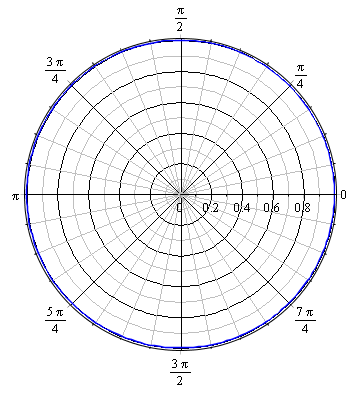The circle

The equation

$$r=2a\cos\theta+2b\sin\theta$$

represents a circle with centre $\left(a,b\right)$ (in Cartesian coordinates) and radius $\sqrt{a^2+b^2}$.

Here is $r=2\cos\theta$, the circle with centre $\left(1,0\right)$ (in Cartesian coordinates) and radius $1$.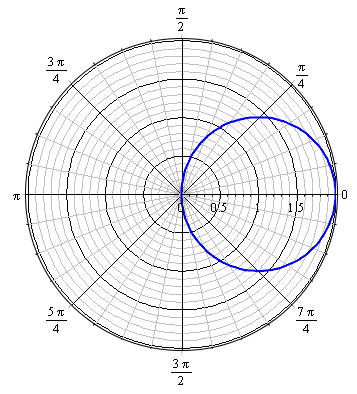The half-line

The equation $\theta = \alpha$ where $\alpha$ is a constant represents a half-line from the origin outwards, with angular coordinate $\alpha$ radians.

Here is the polar coordinate function $\theta = \frac{\pi}{3}$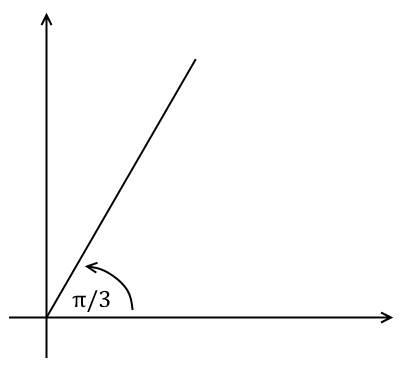The straight perpendicular line

The equation

$$r = \frac{d}{\cos(\alpha-\theta)}$$

represents a straight line at a perpendicular distance $d$ from the origin, so that the perpendicular line drawn from the origin to the straight line makes a fixed angle $\alpha$ with the horizontal axis.

The straight line at a perpendicular distance $d$ from the origin $O$, where the perpendicular line $OA$ makes a fixed angle $\alpha$ with the initial line.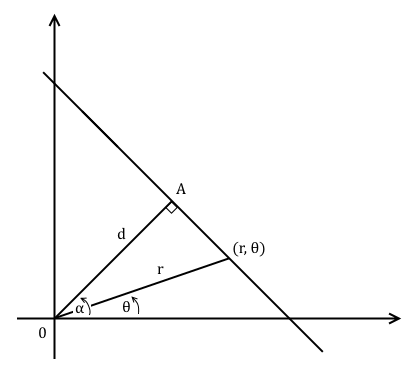For each point $(r,\theta)$ on the straight line you have

$$\cos(\alpha-\theta) = \frac{d}{r} \Rightarrow r = \frac{d}{\cos(\alpha-\theta)}$$

Here is $r=\frac{5}{\cos(\pi/6-\theta)}$ plotted from $\theta=0\ldots\frac{\pi}{4}$.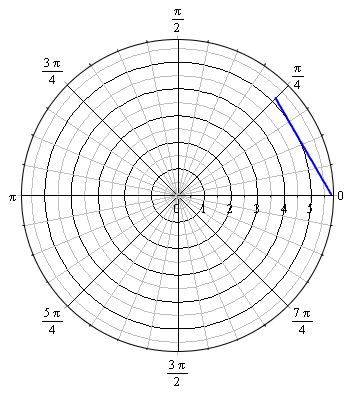The cardioid

The equation

$$r = 2a\left(1+\cos\theta\right)$$

where $a$ is a positive constant, represents a cardioid. Note that the cardioid is an even function (i.e. $r(\theta)=r(-\theta)$) because $\cos$ is an even function.

Here is the cardioid $r=6\left(1+\cos\theta\right)$.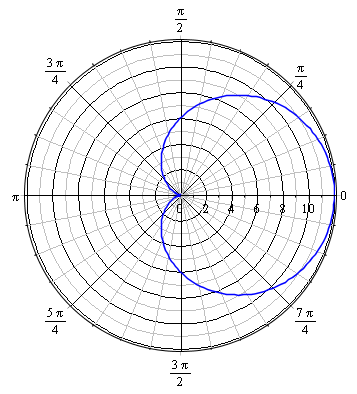Can you guess why it's called a 'cardioid'?

Converting between Cartesian and polar coordinates

In FP2 you will be asked to convert an equation from Cartesian $(x,y)$ coordinates to polar coordinates $(r,\theta)$ and vice versa. To do this you'll need to use the rules

$$x = r\cos\theta ~\textrm{ and }~ y = r\sin\theta$$

as well as

$$r = \sqrt{x^2+y^2}$$

Example

Q) Convert the equation $x^2+y^2 = 4$ from Cartesian coordinates into polar coordinates.

A) Remembering that $r = \sqrt{x^2+y^2}$,

$$r^2 = 4 \Rightarrow r = 2 ~\textrm{ since }~ r \ge 0$$

Example

Q) Convert the equation $xy = 4a^2$ from Cartesian coordinates into polar coordinates.

A) Remembering that $x=r\cos\theta$ and $y=r\sin\theta$,

$$\left(r\cos\theta\right)\left(r\sin\theta\right) = 4a^2$$

$$\Rightarrow r^2 \sin\theta\cos\theta = 4a^2$$

Since $\sin(2\theta) = 2\sin\theta\cos\theta$,

$$r^2 \left(\frac{1}{2}\sin(2\theta)\right) = 4a^2$$

$$\Rightarrow r^2 = \frac{8a^2}{\sin(2\theta)}$$

Example

Q) Convert the equation $r=2a\tan\theta$ from polar coordinates into Cartesian coordinates.

A) Remembering that $r = \sqrt{x^2+y^2}$,

$$\sqrt{x^2+y^2} = 2a \frac{\sin\theta}{\cos\theta}$$

Since $x = r\cos\theta$ and $y = r\sin\theta$

$$\sqrt{x^2+y^2} = \frac{2ay}{x}$$

$$\Rightarrow x^2+y^2 = \frac{4a^2y^2}{x^2}$$

Example

Q) Convert the equation $\theta = \frac{\pi}{3}$ from polar coordinates into Cartesian coordinates.

A) Remembering that $\theta = \tan^{-1}\left(\frac{y}{x}\right)$,

$$\tan^{-1}\left(\frac{y}{x}\right) = \frac{\pi}{3}$$

$$\Rightarrow \frac{y}{x} = \tan\left(\frac{\pi}{3}\right) = \sqrt{3}$$

Therefore $y=\sqrt{3}x$ where $x \ge 0$.

Integrating polar coordinate functions

The area of the region enclosed by the polar coordinate function $r(\theta)$ from $\theta=\alpha$ to $\theta=\beta$ is

$$\int_{\alpha}^{\beta} \frac{1}{2} r^2 \mathop{d\theta}$$

Take some point $P(r,\theta)$ and a nearby point $Q(r+\delta r,\theta+\delta \theta)$ on a curve defined with polar coordinates. A circle centered at the origin and radius $r$ intercepts the straight line from the origin $O$ to $Q$ at some other point $P$. Another circle centered at the origin and radius $r+\delta r$ intercepts the straight line from the origin to $P$. In C1 you learned that the area of a circular sector with radius $r$ and angle $\theta$ (in radians) is $\frac{1}{2}r^2 \theta$. Therefore $$\frac{1}{2}r^2 \delta\theta \lt ~\textrm{area of } OPQ \lt \frac{1}{2}\left(r+\delta r\right)^2\delta\theta$$ Therefore to find the area of a section enclosed by a curve from $\theta=\alpha$ to $\theta=\beta$, you must sum over every infinitesimal sector $$\sum_{\theta=\alpha}^{\beta} \frac{1}{2}r^2 \delta\theta \lt ~\textrm{area of whole sector } \lt \sum_{\theta=\alpha}^{\beta} \frac{1}{2}\left(r+\delta r\right)^2 \delta\theta$$ Since $\lim_{\delta\theta\to 0} \delta r = 0$, $$\sum_{\theta=\alpha}^{\beta} \frac{1}{2}r^2 \delta\theta \to \int_{\alpha}^{\beta}\frac{1}{2}r^2 \mathop{d\theta} ~\textrm{ and } \sum_{\theta=\alpha}^{\beta} \frac{1}{2}\left(r+\delta r\right)^2 \delta\theta \to \int_{\alpha}^{\beta}\frac{1}{2}r^2 \mathop{d\theta}$$ Therefore the area of the whole sector is $$\int_{\alpha}^{\beta}\frac{1}{2}r^2 \mathop{d\theta}$$

Example

Q) Find the area enclosed by $r = \theta$ from $\theta=0$ to $\theta=\frac{\pi}{6}$

A) The area is

$$\int_{\alpha}^{\beta} \frac{1}{2} r^2 \mathop{d\theta} = \frac{1}{2}\int_{0}^{\frac{\pi}{6}} \theta^2 \mathop{d\theta} = \frac{1}{2}\left[\frac{1}{3}\theta^3\right]_{0}^{\frac{\pi}{6}} = \frac{1}{6}\left(\frac{\pi}{6}\right)^3 = \frac{\pi^3}{1296} \textrm{ units}^2$$

Example

Q) Find the area enclosed by $r = 1+\cos\theta$ from $\theta=0$ to $\theta=\frac{\pi}{2}$

A) The area is

\begin{align} \int_{\alpha}^{\beta} \frac{1}{2} r^2 \mathop{d\theta} &= \frac{1}{2}\int_{0}^{\frac{\pi}{2}}\left(1+\cos\theta\right)^2 \mathop{d\theta} \\ &= \frac{1}{2}\int_{0}^{\frac{\pi}{2}}\left(1+2\cos\theta+\cos^2 \theta\right) \mathop{d\theta} \\ &= \frac{1}{2}\int_{0}^{\frac{\pi}{2}}\left(1+2\cos\theta+\frac{1}{2}+\frac{1}{2}\cos(2\theta) \right) \mathop{d\theta} \\ &= \frac{1}{2} \left[\frac{3}{2}\theta +2\sin\theta+\sin(2\theta) \right]_{0}^{\frac{\pi}{2}} \\ &= \frac{1}{2} \left(\frac{3}{2}\left(\frac{\pi}{2}\right)+2+0 \right) \\ &= \left(\frac{3\pi}{8}+1\right) \textrm{ units}^2 \end{align}

Finding tangent lines

You need to know how to find tangent lines parallel and perpendicular to the initial line.

Tangents to curves defined by polar coordinates that are parallel to the initial line (i.e. horizontal) occur where $y = r\sin\theta$ is at a maximum or minimum value, i.e.

$$\frac{d}{d\theta}\left(r\sin\theta\right) = 0$$

Tangents to curves defined by polar coordinates that are perpendicular to the initial line (i.e. vertical) occur where $x = r\cos\theta$ is at a maximum or minimum value, i.e.

$$\frac{d}{d\theta}\left(r\cos\theta\right) = 0$$

Example

Q) For the polar coordinate function $r=\sin\theta$, find the points, if any, where tangents to the curve are parallel and perpendicular to the initial line.

A) Firstly find the points where tangents are parallel to the initial line.

$$\frac{d}{d\theta}\left(r\sin\theta\right) = \frac{d}{d\theta}\left(\sin\theta\sin\theta\right) = \frac{d}{d\theta}\left(\sin^2 \theta\right) = 0$$

Use the chain rule to differentiate this. Let $y=u^2$ where $u=\sin\theta$. Then

$$\frac{d}{d\theta}\left(\sin^2 \theta\right) = \frac{dy}{du}\times \frac{du}{d\theta} = 2u\times \cos\theta = 2\sin\theta\cos\theta$$

Since $\sin(2\theta) = 2\sin\theta\cos\theta$

$$\sin(2\theta) = 0 \Rightarrow \theta = 0,\frac{\pi}{2},\pi$$

Therefore the points are $(0,0)$ and $(1,\frac{\pi}{2})$.

Now to find the points where tangents are perpendicular to the initial line.

$$\frac{d}{d\theta}\left(r\cos\theta\right) = \frac{d}{d\theta}\left(\sin\theta\cos\theta\right) = \frac{d}{d\theta}\left(\frac{1}{2}\sin(2\theta)\right) = \cos(2\theta) = 0$$

Solving for $\theta$

$$\cos(2\theta) = 0 \Rightarrow \theta = \frac{\pi}{4},\frac{3\pi}{4}$$

Therefore the points are $(\frac{1}{\sqrt{2}},\frac{\pi}{4})$, and $(\frac{1}{\sqrt{2}},\frac{3\pi}{4})$.